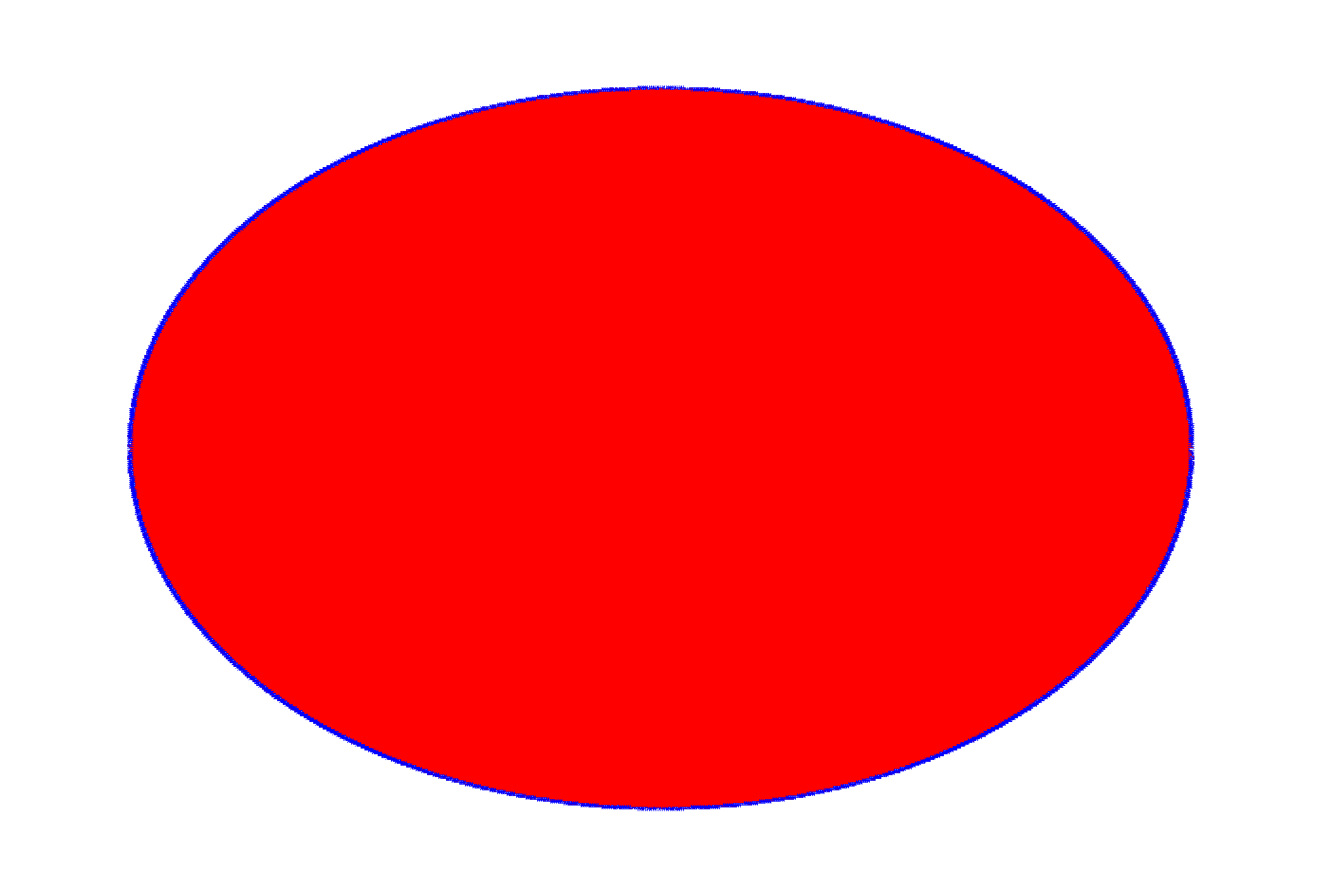ROOT   Reference Guidepolytest1.C File Reference

## Detailed DescriptionThis macro is testing the "compacting" algorithm in TPadPainter.

It reduces the number of polygon's vertices using actual pixel coordinates.It's not really useful, but just to test that the resulting polygon is still reasonable. Initial number of points is 1000000, after "compression" it's 523904 (with default canvas size, before you tried to resize it) - so almost half of vertices were removed but you can still see the reasonable shape. If you resize a canvas to a smaller size, the number of vertices after compression can be something like 5000 and even less. It's easy to 'fool' this algorithm though in this particular case (ellipse is a kind of fringe case, you can easily have a sequence of almost unique vertices (at a pixel level).

//Includes for ACLiC.
#include <cassert>
#include <vector>
#include "TRandom.h"
#include "TCanvas.h"
#include "TError.h"
#include "Rtypes.h"
#include "TNamed.h"
#include "TMath.h"
class PolyTest1 : public TNamed, public TAttLine, public TAttFill {
public:
PolyTest1(unsigned nVertices);
void Paint(const Option_t *notUsed);
void Reset(unsigned nVertices);
private:
enum {
kNPointsDefault = 10000//minimal number of points.
};
std::vector<Double_t> fXs;
std::vector<Double_t> fYs;
};
//_____________________________________________________________
PolyTest1::PolyTest1(unsigned nVertices)
: TNamed("polygon_compression_test1", "polygon_compression_test1")
{
Reset(nVertices);
}
//_____________________________________________________________
void PolyTest1::Reset(unsigned nVertices)
{
//Some canvas must already exist by this point.
//We need a gRandom to exist.
assert(gRandom != 0 && "Reset, gRandom is null");
if (nVertices < kNPointsDefault) {
Warning("Reset", "resetting nVertices parameter to %u", unsigned(kNPointsDefault));
nVertices = kNPointsDefault;
}
fXs.resize(nVertices);
fYs.resize(nVertices);
Double_t xMin = 0., xMax = 0., yMin = 0., yMax = 0.;
assert(xMax - xMin > 0 && yMax - yMin > 0 && "Reset, invalid canvas' ranges");
const Double_t xCentre = xMin + 0.5 * (xMax - xMin);
const Double_t yCentre = yMin + 0.5 * (yMax - yMin);
const Double_t r = TMath::Min(xMax - xMin, yMax - yMin) * 0.8 / 2;
const Double_t angle = TMath::TwoPi() / (nVertices - 1);
for (unsigned i = 0; i < nVertices - 1; ++i) {
const Double_t currR = r + gRandom->Rndm() * r * 0.01;
fXs[i] = xCentre + currR * TMath::Cos(angle * i);
fYs[i] = yCentre + currR * TMath::Sin(angle * i);
}
fXs[nVertices - 1] = fXs;
fYs[nVertices - 1] = fYs;
}
//_____________________________________________________________
void PolyTest1::Paint(const Option_t * /*notUsed*/)
{
}
void polytest1()
{
TCanvas * const cnv = new TCanvas;
cnv->cd();
PolyTest1 * polygon = new PolyTest1(1000000);
polygon->SetLineColor(kBlue);
polygon->SetFillColor(kRed);
polygon->SetLineWidth(1);
polygon->Draw();//Attach a polygon to a canvas.
}
int Int_t
Definition: RtypesCore.h:45
double Double_t
Definition: RtypesCore.h:59
const char Option_t
Definition: RtypesCore.h:66
@ kRed
Definition: Rtypes.h:66
@ kBlue
Definition: Rtypes.h:66
void Warning(const char *location, const char *msgfmt,...)
Use this function in warning situations.
Definition: TError.cxx:231
Option_t Option_t TPoint TPoint const char GetTextMagnitude GetFillStyle GetLineColor GetLineWidth GetMarkerStyle GetTextAlign GetTextColor GetTextSize void char Point_t Rectangle_t WindowAttributes_t Float_t r
Option_t Option_t TPoint TPoint angle
R__EXTERN TRandom * gRandom
Definition: TRandom.h:62
Fill Area Attributes class.
Definition: TAttFill.h:19
virtual void Modify()
Change current fill area attributes if necessary.
Definition: TAttFill.cxx:213
Line Attributes class.
Definition: TAttLine.h:18
virtual void Modify()
Change current line attributes if necessary.
Definition: TAttLine.cxx:245
The Canvas class.
Definition: TCanvas.h:23
Definition: TCanvas.cxx:706
The TNamed class is the base class for all named ROOT classes.
Definition: TNamed.h:29
virtual void Paint(Option_t *option="")
This method must be overridden if a class wants to paint itself.
Definition: TObject.cxx:525
Double_t Rndm() override
Machine independent random number generator.
Definition: TRandom.cxx:552
Short_t Min(Short_t a, Short_t b)
Returns the smallest of a and b.
Definition: TMathBase.h:198
Double_t Cos(Double_t)
Returns the cosine of an angle of x radians.
Definition: TMath.h:592
Double_t Sin(Double_t)
Returns the sine of an angle of x radians.
Definition: TMath.h:586
constexpr Double_t TwoPi()
Definition: TMath.h:44

Definition in file polytest1.C.